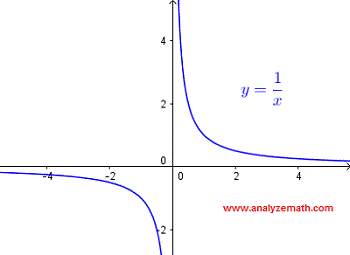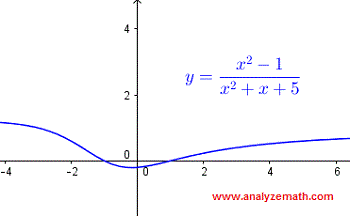# Find The Domain of Rational Functions Questions With Solutions

How to find the domain of rational functions? Examples are presented along with detailed solutions and explanations and also graphical interpretation.

## How to find the domain of a rational function?

We first need to understand that $\dfrac{1}{x}$ takes real values only if the denominator is not equal to 0. In this case $x \ne 0$. This can easily be verified by examining the graph of $y = \dfrac{1}{x}$ shown below: The graph "exists" for all values of $x$ except 0.
The domain in interval form is given by: $(-\infty , 0) \cup (0 , \infty)$### Example 1

Find the domain of the function $f(x) = \dfrac{1}{x - 2}$.
Solution:
The given function takes real values and is therefore real if
$x - 2 \ne 0$ or $x \ne 2$
In interval form, the domain is given by
$(-\infty , 2) \cup (2 , \infty)$
Below is shown the graph of $f$ and we can see that the given function is undefined at x = 2.### Example 2

Find the domain of the function $f(x) = \dfrac{x + 3}{(x - 1)(x + 2)}$.
Solution:
The given function takes real values and is therefore real if
$(x - 1)(x + 2) \ne 0$
The expression (x - 1)(x + 2) is not equal to zero if x ≠ 1 and x ≠ -2.
In interval form, the domain is given by
$(-\infty , - 2) \cup (-2 ,1 ) \cup (1 , \infty)$
Below is shown the graph of $f$ and we can see that the given function is undefined at x = -2 and x = 1.### Example 3

Find the domain of the function $f(x) = \dfrac{1}{ {x^2 - 4}}$.
Solution:
The given function takes real values and is therefore real if
$x^2 - 4 \ne 0$
Factor x 2 - 4 and rewrite the inequality as
$(x - 2)(x + 2) \ne 0$
The expression (x - 2)(x + 2) is not equal to zero if x ≠ -2 and x ≠ 2.
In interval form, the domain is given by
$(-\infty , - 2) \cup (-2 ,2 ) \cup (2 , \infty)$
Below is shown the graph of $f$ and we can see that the given function is undefined at x = -2 and x = 2.### Example 4

Find the domain of the function $f(x) = \dfrac{x + 1}{x^2 + x - 2}$.
Solution:
The given function takes real values and is therefore real if
$x^2 + x - 2 \ne 0$
Factor x 2 + x - 2 and rewrite the inequality as
$(x - 1)(x + 2) \ne 0$
The expression (x - 1)(x + 2) is not equal to zero if x ≠ 1 and x ≠ - 2.
In interval form, the domain is given by
$(-\infty , - 2) \cup (-2 ,1 ) \cup (1 , \infty)$
Below is shown the graph of $f$ and we can see that the given function is undefined at x = -2 and x = 1.### Example 5

Find the domain of the function $f(x) = \dfrac{x^2 - 1}{x^2 + x + 5}$.
Solution:
The given function takes real values and is therefore real if
$x^2 + x + 5 \ne 0$
The expression x 2 + x + 5 cannot be factored over the reals. We therefore need to solve the quadratic equation using the discriminant $\Delta$.
$x^2 + x + 5 = 0$
$\Delta = b^2 - 4 a c = (1)^2 - 4(1)(5) = -19$
The discriminant is negative and therefore no real value of x makes the expression x 2 + x + 5 equal to zero. The domain is the set of all real numbers
In interval form, the domain is given by
$(-\infty , \infty)$
Below is shown the graph of $f$ and we can see that it defined for all values of x real.